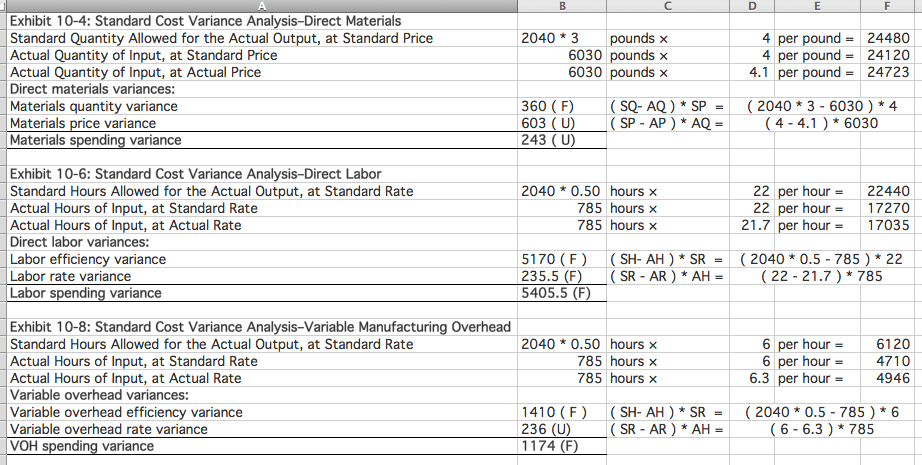# Question & Answer: show FORMULAS FOR EVERY QUESTION MARK YOU SOLVE…..

 Please show FORMULAS FOR EVERY QUESTION MARK YOU SOLVE, Inputs Standard Quantity Standard Price Direct materials 3.0 pounds \$4.00 per pound Direct labor 0.50 hours \$22.00 per hour Variable manufacturing overhead 0.50 hours \$6.00 per hour Actual results: Actual output 2,040 units Actual variable manufacturing overhead cost \$4,946 Actual Quantity Actual price Actual direct materials cost 6,030 pounds \$4.10 per pound Actual direct labor cost 785 hours \$21.70 per hour Enter a formula into each of the cells marked with a ? below Main Example: Chapter 10 Exhibit 10-4: Standard Cost Variance Analysis–Direct Materials Standard Quantity Allowed for the Actual Output, at Standard Price ? pounds × ? per pound = ? Actual Quantity of Input, at Standard Price ? pounds × ? per pound = ? Actual Quantity of Input, at Actual Price ? pounds × ? per pound = ? Direct materials variances: Materials quantity variance ? Materials price variance ? Materials spending variance ? Exhibit 10-6: Standard Cost Variance Analysis–Direct Labor Standard Hours Allowed for the Actual Output, at Standard Rate ? hours × ? per hour = ? Actual Hours of Input, at Standard Rate ? hours × ? per hour = ? Actual Hours of Input, at Actual Rate ? hours × ? per hour = ? Direct labor variances: Labor efficiency variance ? Labor rate variance ? Labor spending variance ? Exhibit 10-8: Standard Cost Variance Analysis–Variable Manufacturing Overhead Standard Hours Allowed for the Actual Output, at Standard Rate ? hours × ? per hour = ? Actual Hours of Input, at Standard Rate ? hours × ? per hour = ? Actual Hours of Input, at Actual Rate ? hours × ? per hour = ? Variable overhead variances: Variable overhead efficiency variance ? Variable overhead rate variance ? Variable overhead spending variance ?